### Strutt Help

Harmonoise Excess Attenuation    1/1,1/3

Strutt|Environmental Noise|Harmonoise Excess Attenuation inserts the combined excess attenuation for a terrain profile into the active row of the worksheet calculated using the Harmonoise EU noise propagation model.

Unlike other environmental calculations in Strutt, Harmonoise excess attenuation is calculated as the cumulative excess attenuation for a full terrain profile (which may include diffraction edges such as noise barriers or natural terrain edges) - i.e. Harmonoise calculates the overall excess attenuation due to ground reflection, diffraction and atmospheric scattering/refraction. The user only has to add the distance loss and any atmospheric absorption loss.

The Harmonoise model is rather theoretically-complicated, but can be summarised as follows:

• Harmonoise calculates the excess attenuation for a single source-receiver path.
Reflections (other than from the ground) are not modelled directly in Harmonoise; rather, image sources/receivers are used to deal with significant reflection paths, which will have their own excess attenuation which will need to be calculated for each path.
• For each path, the user must input the terrain profile of the path as arrays of coordinates x = {x_1,x_2...x_n}, z= {z_1,z_2...z_n} which define the terrain profile consisting of n elements for the propagation path, with the receiver at the end of the nth ground segment.
The source position is assumed to be at x-coordinate x_0=0, and all heights are relative to the ground height at the source - i.e. z_0=0.
A template sheet is provided for Harmonoise calculations which simplifies entry of the ground profile.
• The source and receiver heights (above the local ground plane) z_s and z_r are specified by the user - i.e. the source and receiver coordinates in the global coordinate system are (0,z_s) and (x_n,z_n+z_r)
• The user may also optionally enter the standard deviations sigma_(h_s) and sigma_(h_r) of the source and receiver heights.
For most applications, this 'smears' some of the calculated phase interference effects for ground reflections to reflect a more-realistic result rather than getting a strong phase effect which in practice would only occur for precisely-defined source and receiver heights.

• Diffraction and reflection losses are calculated using both the primary ('true') source/receiver, as well as a number of secondary sources and secondary receivers which are (in general) located at diffraction edges.
Image sources/receivers are denoted by a prime prime - i.e. S prime indicates the image source; R prime is the image receiver
E.g. in the simple case of a single diffraction edge (e.g. a noise barrier), the overall excess attenuation would consist of:
• The diffraction loss from the primary source to the primary receiver via the diffraction edge
• The ground reflected path from the primary source to a secondary receiver located at the diffraction edge
• The ground reflected path from a secondary source located at the diffraction edge to the primary receiver.

• Based on the entered terrain profile, the Harmonoise model splits the excess attenuation into a number of diffraction losses where a vertex blocks the line-of-sight from a source to a receiver, plus ground reflections which are calculated over the terrain intervals between the diffraction edges. A recursive approach is used for finding significant diffraction edges
• Firstly, Harmonoise checks whether there are any edges above the line-of-sight from source S to receiver R.
• If the line-of-sight is blocked, the edge with highest path difference is identified and the diffraction loss of this edge (K) is calculated.
If the line-of-sight is not blocked, the entire terrain profile is calculated using the ground reflection model.
• Harmonoise then checks the terrain between the source to the diffraction edge (S-K) and between the diffraction edge and the receiver (K - R) for any diffraction edges above the lines of sight from S to K or from K to R.
• If diffraction edges are found, the terrain interval is subdivided at the diffraction edge and the search for significant diffraction edges continues until there are no edges above the line-of-sight.

• This results in a set of 'significant diffraction edges', each of which has a diffraction loss associated with it, and a series of intervals between diffraction edges, over which ground effects are calculated.
E.g. in the example below, the overall Harmonoise excess attenuation would consist of:
• Ground effect calculated with the source at the real source (S_r) and the secondary receiver at point P_2.
• A diffraction loss associated with vertex P_2
• Ground effect calculated with a secondary source at P_2 and a secondary receiver at point P_5.
• A diffraction loss associated with vertex P_5
• Ground effect calculated with a secondary source at P_5 and a secondary receiver at point P_6.
• A diffraction loss associated with vertex P_6
• Ground effect calculated with a secondary source at P_6 and the receiver at the real receiver (R_r).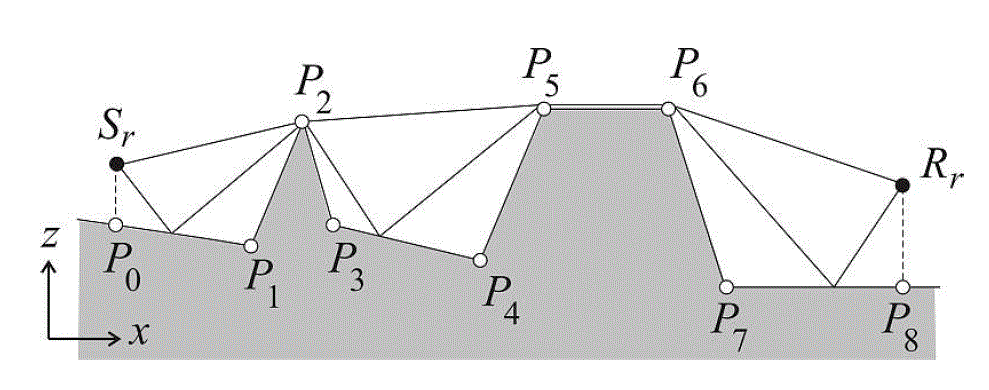• Harmonoise calculates the ground reflection based on the ground impedance of each calculation segment, which is calculated from a user-entered array of flow-resistivity values {sigma_1,sigma_2...sigma_n}. Reflective ground (i.e. infinite impedance) is assigned in Strutt by entering a flow resistivity value of 0 for a segment.
• The overall excess attenuation is calculated as the logarithmic sum of the overall diffraction/ground reflection loss via the terrain profile, plus a scattered component from atmospheric turbulence.
The effect of atmospheric turbulence is to limit the maximum practical excess attenuation to ~25-30 dB (depending on the turbulence parameter, which may be fine-tuned by the user if desired by adjusting the value of the turbulence structure parameter gamma_T).
• The effect of refraction via a sound speed gradient in the atmosphere is taken into account by a coordinate transformation of the {x,z} coordinates of the terrain profile to account for curved propagation paths - i.e. under 'adverse' conditions, the effective propagation height increases, reducing the influence of ground reflection/absorption or diffraction.

The Harmonoise model is described in detail in the various published papers describing the model (see References section at the end). The following sections are a summary of key features of the model.

Diffraction losses in Harmonoise are calculated using the Degout barrier formula, which is described in detail in the Barrier Attenuation calculation.

The path difference delta for diffraction is calculated automatically from the terrain as follows based on the magnitude of the diffraction angle theta = theta_S+theta_R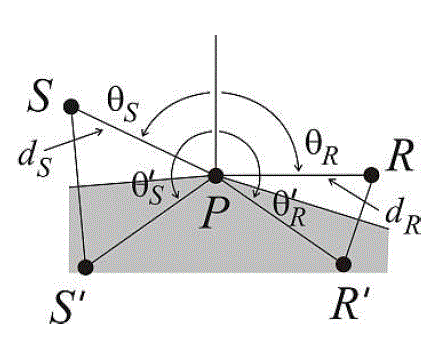• Where theta <= pi: (illuminated zone or grazing attenuation)

delta = -(d_s+d_r-d_d)
d_s,d_r are the distances from the source and receiver to the diffraction vertex
d_d is the direct source-receiver distance; d_d=sqrt(d_s^2+d_r^2-2d_s d_r cos(theta))
• Where theta > pi: (shadow zone)

delta = d_d(1/2 epsilon^2 + 1/3 epsilon^4)
d_d = d_s+d_r
epsilon = sqrt(d_s d_r)/d_d (theta-pi)

Ground attenuation is calculated using a complicated model, which accounts for several propagation factors.

The ground effect is calculated over each terrain segment, and a local coordinate system is used for each segment with the source/receiver heights h_s and h_r calculated by Strutt relative to each segment. These local source heights are used for image source/receiver calculations.

Each segment is characterised as either 'concave' (both local source height h_s and local receiver height h_r are positive), 'hull' (local source and receiver heights are both zero, e.g. segment P_5-P_6 in the example above), or 'convex' (one or both of the local source/receiver heights h_s,h_r are negative).

Two overall models are used, the Concave model, which is used when diffraction is not significant, and the Transition model, which 'blends' ground reflection and illuminated-zone diffraction effects.

Concave Model

The concave model applies for terrain that contain no convex segments, and consists of two calculations - the ground reflection from flat ground, and ground reflection from deep 'valley'-shaped terrain, where the flat ground model becomes unstable. A transition factor F_G is used to 'blend' the two calculation regimes, and also helps to compensate for the omission of higher-order reflection paths from the model for valley-shaped terrain

The ground model uses the concept of Fresnel weightings, which are essentially a geometric weighting of how 'efficient' a ground segment is in contributing to the source-receiver reflection. A frequency-modified version of the weighting is used in the Harmonoise model. Refer below for calculation of Fresnel weightings.

The ground reflection from flat ground is the weighted sum of the reflection from each ground segment, weighted using the modified Fresnel weighting w_k of each segment:

Delta L_(G,flat) = sum w_k Delta L_k

The ground reflection from a single ground segment k is calculated using the following equation:

Delta L_k = 10log_10(|1+C_k D_k Q_k|^2 + (1-C^2)|D_k Q_k|^2)

The first term is for coherent reflection from the ground (i.e. pressure doubling), the second term is for incoherent reflection (i.e. energetic reflection).

C_k is a coherence factor for each ground segment k - i.e. whether the reflection is coherent (C_k=1), incoherent (C_k=0) or intermediate.

D_k is a geometric weighting factor for the reflection strength from segment k, which takes into account the additional path difference, any diffraction loss (e.g. from image sources/receivers) and phase differences associated with the reflection from segment k.

Q_k is the spherical wave reflection coefficient for each ground segment k

The ground reflection from valley-shaped terrain is similar, but is calculated as an overall term for the terrain segment as a whole, rather than as the sum of individual elements:

Delta L_(G,"valley") = 10log_10(|1 + sum w_k C_k D_k Q_k|^2+sum w_k(1-C_k^2)|D_k Q_k|^2)

The overall concave terrain ground reflection is calculated using a transition factor F_G:

Delta L_(G,C) = F_G DeltaL_(G,flat) + (1-F_G)Delta L_(G,"valley")

F_G = 1-e^(-1//x_G^2) is an empirically-tuned transition factor for flat/valley shaped terrain.

x_G = N_w/sqrt(1+(f//f_c)^2) is a non-dimensional frequency transition factor

f_c is a transition frequency (refer below for additional detail)

N_w = sum w_k is effectively a measure of the 'curvature' of the terrain:

• N_W ~~1 indicates near-flat terrain
• N_W < 1 indicates 'convex' terrain where the ground reflection is dispersed away from the receiver
• N_W > 1 indicates 'concave' terrain where the ground reflection is focussed towards the receiver

Transition model The transition model uses a mixture of ground reflection, which is calculated using a modified version of the Concave ground model, and illuminated-zone diffraction, which is calculated using the diffraction model.

If P_i...P_j is the ground segment of points containing at least one convex surface, and P_k is the vertex which has the smallest negative path difference (i.e. closest to 0) - effectively the 'highest point' under the line-of-sight from P_i to P_j, the ground attenuation for the segment P_i...P_j under the transition model Delta L_(G,T) is calculated as follows:

Delta L_(G,T) = chi Delta L_1 + (1-chi)Delta L_2

where:
Delta L_1 = Delta L_D(P_i,P_k,P_j) + Delta L_(G,C)(P_i,P_k)+Delta L_(G,C)(P_k,P_j) is the ground attenuation including diffraction:

Delta L_D(P_i,P_k,P_j) is the illuminated zone diffraction loss for the diffraction edge P_k
Delta L_(G,C)(P_i,P_k) is the ground loss (calculated using the Concave ground model) for the terrain from P_i to the diffraction edge P_k
Delta L_(G,C)(P_k,P_j) is the ground loss (calculated using the Concave ground model) for the terrain from the diffraction edge P_k to the end of the terrain interval at P_j

Delta L_2 = Delta L_(G,C)(P_i,P_j) is the ground loss (no diffraction) for the entire terrain between P_i and P_j

chi is a transition factor accounting for the balance between diffraction and reflection

chi = chi_2 + (1-chi_1)(1-chi_2), where:

chi_1={(1-e^(-1//tau_1^2) " for " tau_1 > 0),(1 " for " tau_1 <= 0) :}

tau_1 = (delta_("diff")-delta_(spek,avg))/(lambda//8) compares the path difference for diffraction to the average terrain height
delta_(spek,avg) = (sum w_k delta_(spek,k))/(sum w_k) is the weighted average of the path difference for reflection delta_(spek,k) for each terrain segment

(Sign convention is that path differences are negative in the illuminated zone, hence delta_("diff") > delta_(spek,avg) because diffraction edge is the 'highest point' of the terrain.)

chi_1 quantifies whether the diffraction is significant relative to the surface roughness of the terrain - by Rayleigh's criterion, if the size of the obstacle causing diffraction is less than lambda//8 compared to the average terrain, then diffraction should be neglected since the object is better characterised as "roughness" of the ground. chi_1 rarr 1 indicates the diffraction edge being insignificantly-small compared to the terrain.

chi_2 = {(1-e^(-1//tau_2^2) " for " tau_2 > 0),(1 " for " tau_2 <= 0) :}

tau_2 = |delta_"diff"|/(lambda//64)

chi_2 is a 'transition' factor for continuity between the illuminated zone and the shadow zone. chi_2 rarr 1 as the diffraction edge approaches the line-of-sight.

Coherence factor C_k accounts for the coherence of the ground-reflected component.

C_k = C_A C_B, where:

C_A = e^(-0.5 sigma_phi^2) accounts for phase fluctuations due to frequency-averaging and uncertainty of the source/receiver position

sigma_phi is the standard deviation of the phase fluctuations between direct and reflected sound
(sigma_phi / phi)^2 = (sigma_f/f)^2[+(sigma_(h_s)/h_s)^2][+(sigma_(h_r)/h_r)^2]

sigma_f/f accounts for integration of the calculation across a frequency band; sigma_f/f = 1/3 (2^(0.5B)-2^(-0.5B)) where B=1 for octave-bands, B=1/3 for 1/3-octave bands.

sigma_(h_s) and sigma_(h_r) are the standard deviations of the source/receiver heights (input parameters to the model). The sigma_(h_s) term is only considered for the primary source (i.e. not included when calculating from a secondary source at a diffraction edge); similarly the sigma_(h_r) term is only included for the primary receiver

phi is the phase difference for the reflection.
Where the path difference of the reflection delta_(spek,k) is known, phase is calculated as phi = k delta_(spek,k)
Otherwise, the phase is approximated by phi ~~ (2 pi f)/c_0 (2 h_s h_r)/d_(SR)

C_B = e^(-0.375 D_T gamma_T k^2 rho^(5//3) d_(SR)) accounts for phase differences due to fluctuations of the sound speed and atmospheric turbulence

D_T is a constant (0.364)
gamma_T is a turbulence structure parameter for wind speed and temperature fluctuations:
gamma_T = (C_T/T)^2 +22/3 (C_W/c_0)^2; however in practice the turbulence parameters C_T and C_W are not usually known, and it is more common to specify values of gamma_T as a tuneable input to the model.
Strutt uses a default value of gamma_T = 5*10^-6 as recommended by Harmonoise for "moderate turbulence".
rho = (h_s h_r)/(h_s+h_r) is half of the mean separation of the direct and reflected ray paths. rho=0 for the case of a hull segment.

For convex segments, C_k is calculated as above, except using the absolute value |h_s|,|h_r| of the source receiver heights.

Geometric weighting factor D_k accounts for the strength of the reflected sound relative to the direct sound. At its simplest (a ground reflection with no diffraction), it simply accounts for the additional propagation distance associated with the reflection.

D_k is calculated for the following geometric scenarios:

• Case 1 No Diffraction:

D_(k,1) = (p_f (S prime,R))/(p_f (S,R)), where:

p_f \ (d) = (e^(jkd))/(d)  is the free-field sound pressure for a path of distance d.

D_(k,1) is essentially the ratio of distances for the reflected path relative to the direct path.
• Case 2 Primary Source to Secondary Receiver:

D_(k,2) = (p_d (S prime,P_j,R))/(p_d (S,P_j,R)), where:

P_j is the diffraction edge (secondary receiver)

p_d (d) = (e^(jkd))/(d) 10^((Delta L_D)/20) is the diffracted sound pressure of a diffraction path with path distance d and diffraction loss Delta L_D

D_(k,2) is essentially the relative strength of the image source S prime at the receiver R via diffraction edge P_j - i.e. the ground reflection from the source diffracted over the intermediate barrier.
(N.B. there appears to be a typo in the 2011 Harmonoise paper: the D_(k,2) term uses p_f in the denominator rather than p_d. Strutt uses p_d as per the 2007 Harmonoise paper as this makes more physical sense.)
• Case 3 Secondary Source to Primary Receiver:

D_(k,3) = (p_d (S ,P_i,R prime))/(p_d (S ,P_i,R)), where:

P_i is the diffraction edge (secondary source)

D_(k,3) is essentially the relative strength of the source S at the image receiver R prime via diffraction edge P_i - i.e. the ground reflection from the diffraction source at the intermediate barrier.
• Case 4 Secondary Source to Secondary Receiver:

D_(k,4) = (p_d (S ,P_i,P_j prime))/(p_d (S ,P_i,P_j))(p_d (P_i prime ,P_j,R))/(p_d (P_i ,P_j,R )), where:

P_i is the first diffraction edge (secondary source)

P_j is the second diffraction edge (secondary receiver)

D_(k,4) accounts for the various image source and image receiver paths between the secondary source and the secondary receiver
The geometric terms D_k given above strictly only apply to concave or hull ground segments - i.e. for a convex segment (because either the source or receiver height below the segment is negative) an image reflection is not geometrically possible.
However, ground reflection from convex surfaces is still included in the Harmonoise model, by multiplying the D_k terms for convex segments by (p_d (S,X,R prime))/(p_d (S,X,R)), where X is the point where the line of sight from S-R intersects the ground plane of the convex element (i.e. the 'imaginary' specular reflection point for image source S prime):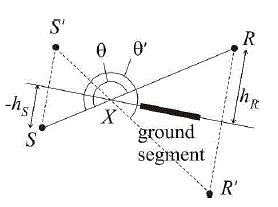In effect, for convex segments, Harmonoise calculates the ground reflection from a diffracted ray that 'bends' around point X to reflect off the convex segment, and the D_k term is reduced in strength to account for the additional diffraction.

Spherical reflection coefficient Q_k is calculated using a modified version of the classical theory for reflection of sound above a ground plane (the Weyl-Van der Pol equation) based on the work of Chien and Soroka:

Q_k = R_p + (1-R_p)(F_Q)^(n_G), where:

R_p is the plane-wave reflection coefficient (Zcos(theta)-Z_0)/(Zcos(theta)+Z_0)

Z is the impedance of the ground plane, calculated from the flow resistivity sigma using the Delaney and Bazley theory for oblique incidence as described in the Porous Absorber calculation)

Z_0 is the characteristic acoustic impedance (rho_0 c_0) of air

The second term (1-R_p)(F_Q)^(n_G) in the spherical wave coefficient is known as the ground wave term, and corrects the plane wave coefficient R_p for the interaction of a curved wavefront and the ground plane

F_Q is known as the boundary loss factor and is given (analytically) by:

F_Q (w) = 1+j sqrt(pi) w e^(-w^2)"erfc"(-jw), where "erfc"(x) is the complimentary error function

w = 0.5 (1+j) sqrt(k d_(S prime R))(cos(theta)+1/Z) is a complex numerical distance term: w = w_r+jw_i

d_(S prime R) is the distance of the reflected path (image source-receiver)

Evaluating F_Q analytically is too mathematically-intensive, and therefore Strutt uses an approximate form to calculate F_Q:

• For short-distances (w_r < 3.9 or w_i < 3), Strutt uses the Matta and Reichel formula:
e^(-w^2)"erfc"(-jw)=K_1+jK_2, where:

K_1 = (h_m w_i)/(pi(w_r^2+w_i^2))+(2 w_i h_m)/pi sum_(n=1)^oo (e^(-n^2 h_m^2)(w_i^2+w_r^2+n^2 h_m^2))/((w_i^2-w_r^2+n^2 h_m^2)+4 w_i^2 w_r^2)-w_i/pi E(h)+n_p P

h_m is the average propagation height h_m = (h_s+h_r)/2

n_p = {(1 " if " w_i < pi//h_m),(0.5 " if " w_i = pi//h_m),(0 " if " w_i > pi//h_m) :}

P = 2e^-(w_r^2+(2 w_i pi//h_m)-w_i^2)[(AC-BD)//(C^2+D^2)]

K_2 = (h_m w_r)/(pi(w_r^2+w_i^2))+(2 w_r h_m)/pi sum_(n=1)^oo (e^(-n^2 h_m^2)(w_i^2+w_r^2-n^2 h_m^2))/((w_i^2-w_r^2+n^2 h_m^2)+4 w_i^2 w_r^2)-w_r/pi E(h)-n_q Q

n_q = {(1 " if " w_i < pi//h_m),(0.5 " if " w_i = pi//h_m),(0 " if " w_i > pi//h_m) :}

Q = 2e^-(w_r^2+(2 w_i pi//h_m)-w_i^2)[(AD+BC)//(C^2+D^2)]

A = cos(2 w_r w_i)
B = sin(2 w_r w_i)
C = e^(-2 w_i pi//h_m)-cos(2 w_r pi//h_m)
D = sin(2 w_r pi//h_m)
E(h) <= (2sqrt(pi)e^(-pi^2//h_m^2))/(1-e^(-pi^2)//h_m^2 is the error bound for e^(-w^2)"erfc"(-jw). Attenborough suggests that taking the sum of the first 5 terms of the infinite sums in K_1 and K_2 gives acceptable error for average propagation heights h_m of 0.8m or higher.

• For intermediate-distances (3.9 <= w_r < 6 or 3 < w_i <= 6), Strutt uses the full Abramowitz and Stegun formula:

e^(-w^2)"erfc"(-jw)=jw((0.461315)/(w^2-0.1901635) +(0.09999216)/(w^2-1.7844927) + (0.002883894)/(w^2-5.5253437))

• For long-distances (w_r >= 6 or w_i >= 6), Strutt uses the short-form Abramowitz and Stegun formula:

e^(-w^2)"erfc"(-jw)=jw((0.5124242)/(w^2-0.2752551) +(0.05176536)/(w^2-2.724745) )
Harmonoise modifies the boundary loss factor F_Q to be more-realistic for situations where the sound is close to grazing incidence via term n_G:
n_G = 1-0.7e^(-h_m/(lambda//32))

Note that Q_k is calculated as if for an infinite-sized reflecting surface. Finite-sized surface effects are taken into account via the use of Fresnel weightings w_k for each segment.

For convex segments, Q_k is calculated as above, except using the absolute value |h_s|,|h_r| of the source receiver heights.

The Fresnel weightings, w_k are used to account for the "efficiency" with which a surface can reflect from the source to the receiver.

The Fresnel weightings are based on the Fresnel ellipsoid, which has the image source S prime and the receiver R as its two focal points: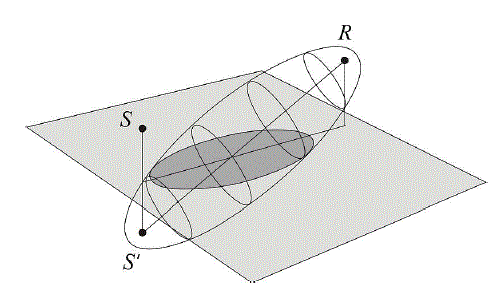The ellipsoid is also defined by the Fresnel parameter n_F which is proportional to the wavelength of sound, and effectively defines the locus of points that can reflect efficiently to the receiver point. A frequency-dependent value of n_F is used in Harmonoise (based on validation studies), which helps to account for phenomena such as surface roughness/scattering that decrease the strength of the ground-reflected component at high frequency.

The intersection between the Fresnel ellipsoid and the ground plane of the segment describes an elliptical region, the Fresnel ellipse. A reflection in Harmonoise is only considered significant if it occurs within the Fresnel ellipse.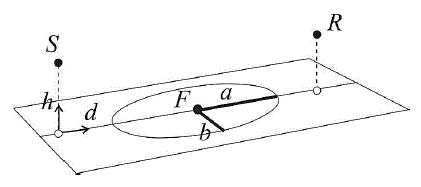.
Note that the centre of the Fresnel ellipse is not coincident with the specular reflection point.

The centre of the ellipse occurs at a distance d_F from the projection of the source to the ground plane, and the semi-major axis of the ellipse is a:
d_F = d_(SR)/2(1+(h_s^2-h_r^2)/(D^2-d_(SR)^2))

a = 1/2 sqrt((D^4+(D_S^2-D_R^2)^2-2D^2(D_S^2+D_R^2))/(D^2-d_(SR)^2))

where:
D = lambda/n_F + sqrt((h_S+h_R)^2+d_(SR)^2)

D_S^2=d_F^2+h_S^2

D_R^2=(d_(SR)^2-d_F^2)+h_R^2

These paraneters are used to calculate the unmodified Fresnel weightings w_(F,k) for each ground segment, using n_F=lambda//8 for the unmodified weighting. The unmodified Fresnel weighting w_(F,k) is the proportion of the surface that lies within the Fresnel ellipse (i.e. the proportion of the surface that can efficiently-reflect to the receiver):

w_(F,k) = F_w (xi_2)-F_w (xi_1), where:

F_w (x)= {(0 " for " x <= -1),(1-1/pi (cos^-1(x)-x sqrt(1-x^2)) " for " -1 < x < 1),(1 " for " x >= 1) :}
xi is a non-dimensional distance within the Fresnel ellipse: xi_m = (d_m-d_F)/a, i.e. |xi_m| > 1 indicates a distance outside of the Fresnel ellipse.
xi_1 and xi_2 are the xi-coordinates of the beginning and end of the terrain segment, respectively.
For continuity, F_w = 0 at the source and F_w = 1 at the receiver.

The unmodified Fresnel weightings do not provide accurate results at high frequency, and therefore Harmonoise uses the modified Fresnel weighting, w_k in calculations. (The unmodified weightings are, however, used as intermediate steps in some calculations.)
The modified Fresnel weighting is centred around the specular reflection point, and uses a frequency-dependent value of n_F:

n_(F,mod)=32(1-e^((f//f_c)^2))
f_c is the transition frequency (see below).

The modified weightings use a modified version of the non-dimensional distance:

w_k = F_w (xi prime_2) - F_w (xi prime_1), where:

xi prime_m = (xi_m-xi_C)/(1-xi_m xi_c)

xi_C = (d_C-d_F)/a

d_C = alpha(f)d_F+[1-alpha(f)]d_(SP)

d_(SP) = d_(SR) h_S/(h_S+h_R) is the distance to the specular reflection point

alpha(f)=[1+(f/f_c)^2]^-1

Transition frequency f_c is used to divide the calculation into high-frequency and low-frequency regimes, with frequency-dependent parameters such as F_G, n_F and alpha (f) being calculated based on the ratio of the band centre frequency f and f_c.
f_c = sqrt(f_(min) f_(max))

f_(min) and f_(max) are based on the phase difference between the direct and reflected sound and are defined as follows:

phi_(max) (f_(min))=pi/2

phi_(max) (f_(max))=pi

where, for each frequency:

phi_(max) is the maximum value of the phase difference phi_k of all the terrain segments k over the calculation interval.

phi_k = "Im"(Q_k)+k*delta_(spek,k)

i.e. the phase change associated with the ground reflection (the imaginary part of the reflection coefficient Q_k) and the phase change associated with the additional path difference delta_(spek,k) between direct and reflected sound).
In evaluating phi_k, segments with zero unmodified Fresnel weighting w_(F,k) and/or convex segments are excluded.

In general, phi_(max) equal to pi or pi/2 will not occur precisely at the centre band frequency f used for calculation. This means that f_(max) and f_(min) are determined as follows:

• The lowest frequency band f_n for which the phase difference phi_k >= pi/2 (for f_(min)) or where phi_k >= pi (for f_(max)) is determined.
• f_(min) and f_(max) are determined by interpolation:

f_min = f_(n-1)+(f_n-f_(n-1))(pi/2-phi(f_(n-1)))/(phi(f_n)-phi(f_(n-1))

f_max = f_(n-1)+(f_n-f_(n-1))(pi-phi(f_(n-1)))/(phi(f_n)-phi(f_(n-1))

Atmospheric scattering is calculated as follows:

Delta L_(scat) = 25 + 10log_10 gamma_T + 3 log_10 f/1000 + 10 log_10 d_(SR)/100

The effect of atmospheric scattering is to limit the practical maximum excess attenuation via the ground profile (i.e. screening or ground absorption) by scattered sound "short-circuiting" the diffraction/reflection paths.

Refraction of sound waves is accounted for by a coordinate transformation of the terrain profile - in effect 'straightening out' the curved ray paths to an equivalent geometry with straight ray paths. The Harmonoise excess attenuation is then calculated using the "neutral-atmosphere" method described above using the new coordinate system.

The coordinate transformation is made in the complex plane. If the original set of coordinates are (in Cartesian) form y = x + jz, the mapping transforms to an equivalent coordinate system dot y= dot x+ j dot z

Harmonoise assumes a linear vertical sound speed profile c(z) = c_0(1+z/R_c), where R_c is the approximate radius of curvature of the curved ray paths.

The coordinate transformation results in a transformed system with approximately-constant sound speed. The assumptions behind the coordinate transformation and the approximation of constant sound speed are valid only for |R_c|>5d_(SR). The new coordinates dot x and dot z are given by the following equations:

dot x = (C_0^2 ddot x)/(ddot x^2+(C_0+ddot z)^2

dot z = (C_0(ddot x^2+ddot z^2+ddot z C_0))/(ddot x^2+(C_0+ddot z)^2)

ddot x = x - (x_0+x_N)/2

ddot z = z-(z_0+z_s+z_n+z_r)/2

C_0=2 ((z_s+z_r)/2+R_C)

Recall that the source and receiver coordinates in the global coordinate system are (x_0,z_s) and (x_n,z_n+z_r) and that Strutt assumes z_0=0 (all heights are relative to terrain at the source point).

The overall Harmonoise excess attenuation is calculated as:

A_(excess) = 10log_10(10^((sum Delta L_D + sum Delta L_G)//10)+10^(Delta L_"scat"//10))
(i.e. the sum of all diffraction and ground reflection terms, logarithmically added with the scattered sound level.

References:

• Van Maercke, D and Defrance J (2007) Development of an Analytical Model for Outdoor Sound Propagation Within the Harmonoise Project, Acta Acustica united with Acustica 93, pp201-212
• Salomons E, Van Maercke D, Defrance J and de Roo F (2011) The Harmonoise Sound Propagation Model, Acta Acustica united with Acustica 97, pp62-74
• Attenborough K, Li KM and Horoshenkov K (2007) Predicting Outdoor Sound, Taylor and Francis, Chapter 2, pp35-39